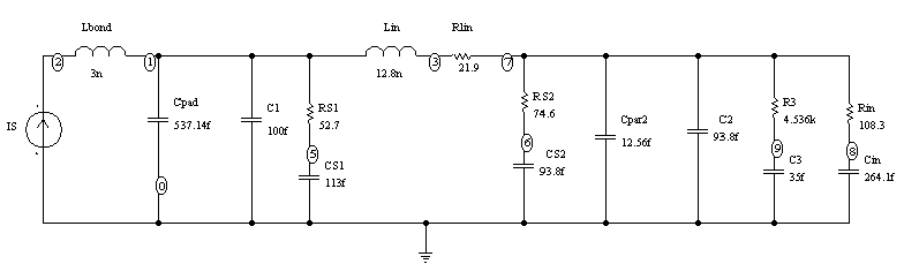# GA-Spice Example:  RF Matching Network## Circuit Topology Description

• C1 and C2 are tuning capacitors
• Lbond models bond wire capacitance
• Lin is an IC inductor
• RS1, CS1, RS2, CS2, and Rin model parasitics of IC inductor
• Cpar2 models metal trace capacitance
• R3 and C3 are the input impedance of a biasing circuit
• Rin and Cin model the input impedance of a MOSFET.

## Problem Description

• Determine the values of C1 and C2 to achieve an input impedance of 50 + j 0 ohms at 2.45 GHz.
• C1& C2 encoded as problem variables using 10 bits each.
• Solve time approximately 15 minutes on a 200 MHz Pentium II notebook computer.

## Results

• Zin = 50.75 - j 3.5 Ohms at 2.45 GHz
• C1 = 306 pf
• C2 = 20 pf

If you would like more details on this problem or further information on the GA-Spice program please contact Dr. Ross.

Home

Last updated March 10, 2020.